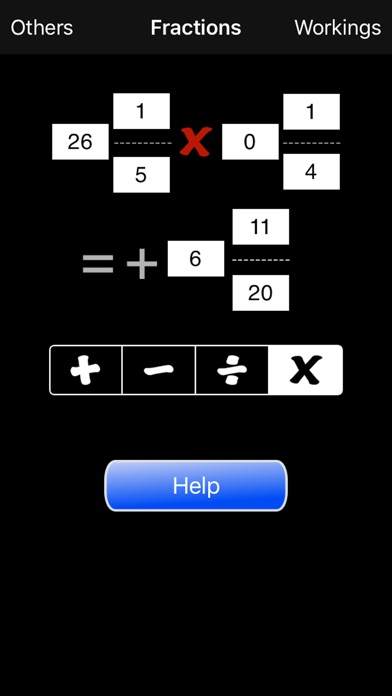Fractions Calculator

iOS UniversalEducation

\$0.99

Do you struggle to work out fractions? Do you need help in understanding how to add, subtract, divide and multiply fractions? Well if you do then this App is for you. Not only does it calculate the answers for you it also comprehensively explains how the answer was arrived at. Along the way the App explains about the Least Common Denominator, proper and improper fractions, simplifying fractions and other general concepts.

New Version: Added negative numbers. Fractions can be negative by specifying the whole part as negative. If the whole part is zero just put a minus sign.

How to use.

Just type in the fractions you want using the keypad. Select any of the editable fields and the keypad appears. The field being editing is shown with a yellow background. You can change more than one number at a time by selecting each field and typing the number. When you have set the fractions as you want then select 'OK'. The keypad is dismissed and the answer is shown.

Use the four calculation buttons (add, subtract, divide and multiply) to select the type of action.

Select the 'Workings' button to see how the result was calculated. This page has sections that explain how the whole number parts are handled, finding the lowest common denomitor, the greatest common factor, how adding, subtracting, dividing and multiplying work and how fractions are simplified.

What's New

Updated UI to handle larger screens better

•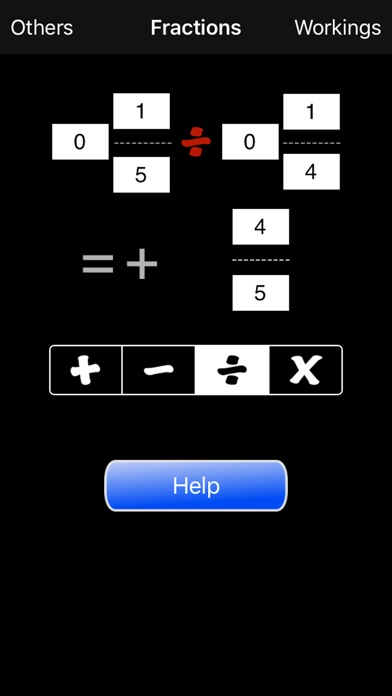•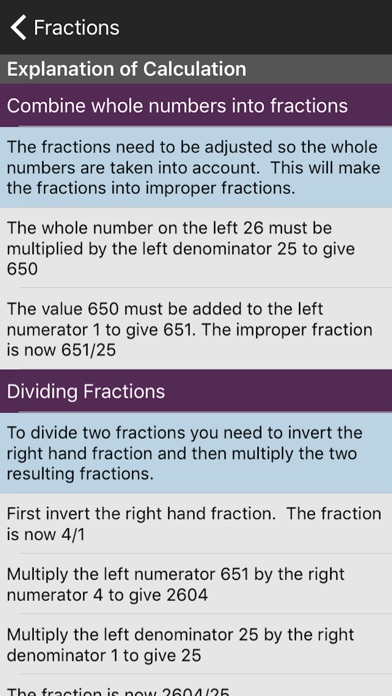•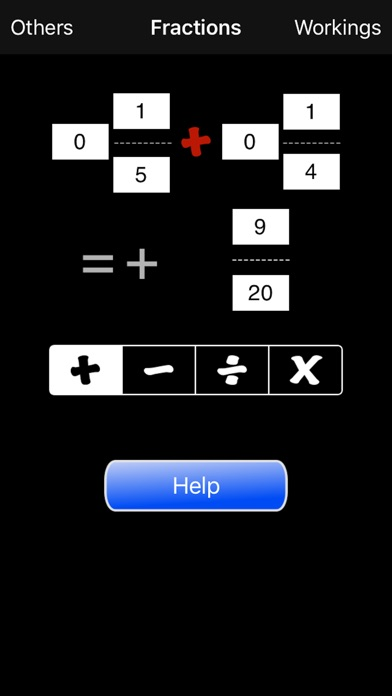•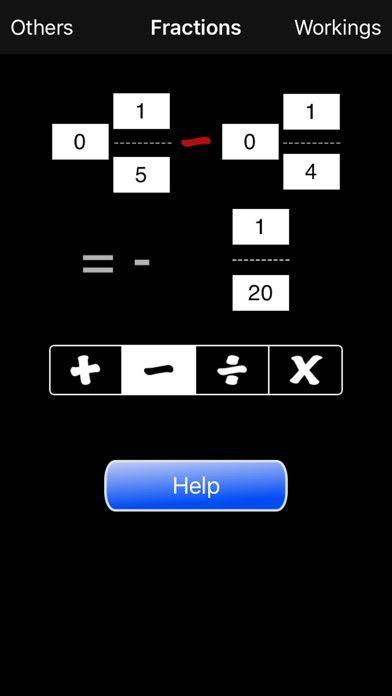•